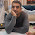Problem: Imagine you've a large database of email-addresses or you copied a web page containing e-mail addresses and other unnecessary text and you want to highlight valid or extract valid E-mail addresses from them.

Solution:
Rules:
i) As per the problem, designing a function(UDF) would be the best approach as it can be used in worksheet as well as sub-routines.
ii) Since it's a email address, @ must appear exactly once.
iii) It must only contain alphanumeric set, underscore(_),hyphen or dash(-) and period/dot(.)
iv) Leftmost and Rightmost character should not be .
v) Period/dot (.) must appear at least once after the @.
vi) There should be either 2 or 3 characters(eg. com, net, org, in, us, uk etc) after the last period (.)
vii) There must be at least one alphanumeric character before @

Function Code:

Dim sArray As Variant, sItem As Variant
Dim n As Long, c As String
'Find the number of @, it should be exactly one.
If n <> 1 Then CheckEmail = False: Exit Function
ReDim sArray (1 To 2)
For Each sItem In sArray
'There should be atleast one character before @.
If Len(sItem) <= 0 Then CheckEmail = False: Exit Function
For n = 1 To Len(sItem)
c = LCase(Mid(sItem, n, 1))
'It must not contain any special character but only alphanumeric, underscore, period and dash or hyphen.
If InStr("abcdefghijklmnopqrstuvwxyz_-.", c) <= 0 And Not IsNumeric(c)  Then CheckEmail = False: Exit Function
Next
'Extreme characters must not be period or dot (.)
If Left(sItem, 1) = "." Or Right(sItem, 1) = "."  Then CheckEmail = False: Exit Function
Next
'There must be atleast one period or dot after @
If InStr(sArray(2), ".") <= 0  Then CheckEmail = False: Exit Function
'After the last dot or period, there must be either exactly 2 or 3 characters.
n = Len(sArray(2)) - InStrRev(sArray(2), ".")
If n <> 2 And n <> 3  Then CheckEmail = False: Exit Function
'It must not contain 2 or more consecutive periods or dots.
If InStr(EmailAddress, "..") > 0  Then CheckEmail = False: Exit Function
CheckEmail = True
End Function